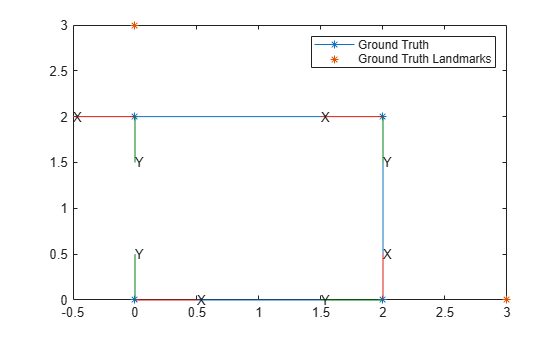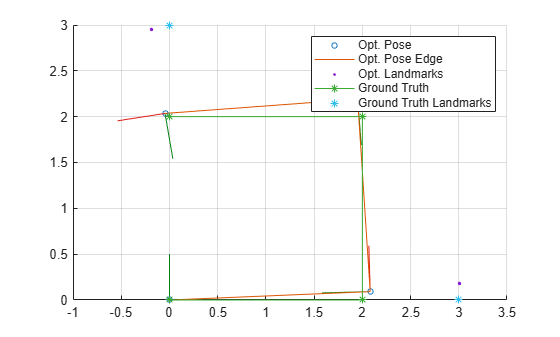# factorGraph

Bipartite graph of factors and nodes

## Description

A `factorGraph` object stores a bipartite graph consisting of factors connected to variable nodes. The nodes represent the unknown random variables in an estimation problem, and the factors represent probabilistic constraints on those variables, derived from measurements or prior knowledge. Add factors and nodes to the factor graph by using the `addFactor` function.

## Creation

### Syntax

``G = factorGraph``

### Description

example

````G = factorGraph` creates an empty `factorGraph` object.```

## Properties

expand all

This property is read-only.

Number of nodes in the factor graph, specified as a positive integer.

This property is read-only.

Number of factors in the factor graph, specified as a positive integer.

## Object Functions

 `addFactor` Add factor to factor graph `fixNode` Fix or free node in factor graph `hasNode` Check if node ID exists in factor graph `isConnected` Check if factor graph is connected `isNodeFixed` Check if node is fixed `nodeIDs` Get all node IDs in factor graph `nodeState` Get or set node state in factor graph `nodeType` Get node type of node in factor graph `optimize` Optimize factor graph

## Examples

collapse all

Create and optimize a factor graph with custom solver options.

Create Factor Graph and Solver Settings

Create a factor graph and solver options with custom settings. Set the maximum number of iterations to `1000` and set the verbosity of the `optimize` output to `2`.

```G = factorGraph; optns = factorGraphSolverOptions(MaxIterations=1000,VerbosityLevel=2)```
```optns = factorGraphSolverOptions with properties: MaxIterations: 1000 FunctionTolerance: 1.0000e-06 GradientTolerance: 1.0000e-10 StepTolerance: 1.0000e-08 VerbosityLevel: 2 TrustRegionStrategyType: 1 ```

Create a GPS factor with node identification number of `1` with NED ReferenceFrame and add it to the factor graph.

```fgps = factorGPS(1,ReferenceFrame="NED"); addFactor(G,fgps);```

Optimize Factor Graph

Optimize the factor graph with the custom settings. The results of the optimization are displayed with the level of detail depending on the `VerbosityLevel.`

`optimize(G,optns);`
```iter cost cost_change |gradient| |step| tr_ratio tr_radius ls_iter iter_time total_time 0 0.000000e+00 0.00e+00 0.00e+00 0.00e+00 0.00e+00 1.00e+04 0 4.20e-05 2.45e-04 Terminating: Gradient tolerance reached. Gradient max norm: 0.000000e+00 <= 1.000000e-10 Solver Summary (v 2.0.0-eigen-(3.3.4)-no_lapack-eigensparse-no_openmp-no_custom_blas) Original Reduced Parameter blocks 1 1 Parameters 7 7 Effective parameters 6 6 Residual blocks 1 1 Residuals 3 3 Minimizer TRUST_REGION Sparse linear algebra library EIGEN_SPARSE Trust region strategy DOGLEG (TRADITIONAL) Given Used Linear solver SPARSE_NORMAL_CHOLESKY SPARSE_NORMAL_CHOLESKY Threads 1 1 Linear solver ordering AUTOMATIC 1 Cost: Initial 0.000000e+00 Final 0.000000e+00 Change 0.000000e+00 Minimizer iterations 1 Successful steps 1 Unsuccessful steps 0 Time (in seconds): Preprocessor 0.000203 Residual only evaluation 0.000000 (0) Jacobian & residual evaluation 0.000014 (1) Linear solver 0.000000 (0) Minimizer 0.003924 Postprocessor 0.000009 Total 0.004136 Termination: CONVERGENCE (Gradient tolerance reached. Gradient max norm: 0.000000e+00 <= 1.000000e-10) ```

Create a matrix of positions of the landmarks to use for localization, and the real positions of the robot to compare your factor graph estimate against. Use the `exampleHelperPlotPositionsAndLandmarks` helper function to visualize the landmark points and the real path of the robot..

```landmarks = [0 -3 0; 3 4 0; 7 1 0]; realpos = [0 0 0; 2 -2 0; 5 3 0; 10 2 0]; exampleHelperPlotPositionsAndLandmarks(realpos,landmarks)```Use Landmarks and Other Data as Factors

Create a factor graph, and add a prior factor to loosely fix the start pose of the robot by providing an estimate pose.

```fg = factorGraph; rng(2) pf = factorPoseSE3Prior(1,Measurement=[0 0 0 1 0 0 0]); addFactor(fg,pf);```

Create `factorPoseSE3AndXYZ` landmark factor objects that relate the first and second pose nodes to the first landmark point, and then add the landmark factors to the factor graph. The landmark factors used here are for SE(3) state space but the process is identical for landmark factors for SE(2) state space. Add some random number to the relative position between the landmark and the ground truth position to simulate real sensor measurements.

```% Landmark 1 Factors measurementlmf1 = landmarks(1,:) - realpos(1,:) + 0.1*rand(1,3); measurementlmf2 = landmarks(1,:) - realpos(2,:) + 0.1*rand(1,3); lmf1 = factorPoseSE3AndPointXYZ([1 5],Measurement=measurementlmf1); lmf2 = factorPoseSE3AndPointXYZ([2 5],Measurement=measurementlmf2); addFactor(fg,lmf1); addFactor(fg,lmf2);```

Create landmark factors for the second and third landmark points, as well, relating them to the second and third pose nodes and third and fourth pose nodes, respectively. Use the `exampleHelperAddNoiseAndAddToFactorGraph` helper function to add noise to the measurement for each landmark factor and add the factors to the factor graph. Once you have added all landmark factors to the factor graph, the IDs of the pose nodes are 1, 2, 3, and 4, and the IDs of the landmark nodes are 5, 6, and 7.

```% Landmark 2 Factors lmf3 = factorPoseSE3AndPointXYZ([2 6],Measurement=landmarks(2,:)-realpos(2,:)); lmf4 = factorPoseSE3AndPointXYZ([3 6],Measurement=landmarks(2,:)-realpos(3,:)); % Landmark 3 Factors lmf5 = factorPoseSE3AndPointXYZ([3 7],Measurement=landmarks(3,:)-realpos(3,:)); lmf6 = factorPoseSE3AndPointXYZ([4 7],Measurement=landmarks(3,:)-realpos(4,:)); exampleHelperAddNoiseAndAddToFactorGraph(fg,[lmf3 lmf4 lmf5 lmf6])```

Use relative pose factors to relate consecutive poses, and add the factors to the factor graph. To simulate sensor readings for the measurements of each factor, take the difference between a consecutive pair of ground truth positions, append a quaternion of zero, and add noise.

```zeroQuat = [1 0 0 0]; rp1 = factorTwoPoseSE3([1 2],Measurement=[realpos(2,:)-realpos(1,:) zeroQuat]); rp2 = factorTwoPoseSE3([2 3],Measurement=[realpos(3,:)-realpos(2,:) zeroQuat]); rp3 = factorTwoPoseSE3([3 4],Measurement=[realpos(4,:)-realpos(3,:) zeroQuat]); exampleHelperAddNoiseAndAddToFactorGraph(fg,[rp1 rp2 rp3])```

Optimize Factor Graph

Optimize the factor graph with the default solver options. The optimization updates the states of all nodes in the factor graph, so the positions of vehicle and the landmarks update.

```fgso = factorGraphSolverOptions; optimize(fg,fgso)```
```ans = struct with fields: InitialCost: 71.6462 FinalCost: 0.0140 NumSuccessfulSteps: 5 NumUnsuccessfulSteps: 0 TotalTime: 0.0328 TerminationType: 0 IsSolutionUsable: 1 ```

Visualize and Compare Results

Get and store the updated node states for the vehicle and landmarks and plot the results, comparing the factor graph estimate of the robot path to the known ground truth of the robot.

`fgposopt = [fg.nodeState(1); fg.nodeState(2); fg.nodeState(3); fg.nodeState(4)]`
```fgposopt = 4×7 -0.0000 0.0000 -0.0000 1.0000 -0.0000 0.0000 0.0000 2.0529 -2.0006 0.0528 0.9991 0.0415 0.0115 0.0053 5.0501 3.0537 0.3775 0.9980 0.0569 0.0210 0.0197 10.0939 2.3060 0.0984 0.9883 0.1423 0.0465 0.0274 ```
`fglmopt = [fg.nodeState(5); fg.nodeState(6); fg.nodeState(7)]`
```fglmopt = 3×3 0.0753 -2.9889 0.0527 3.0294 4.0345 0.5962 7.1825 1.2102 0.1122 ```
`exampleHelperPlotPositionsAndLandmarks(realpos,landmarks,fgposopt,fglmopt)`Dellaert, Frank. Factor graphs and GTSAM: A Hands-On Introduction. Georgia: Georgia Tech, September, 2012.